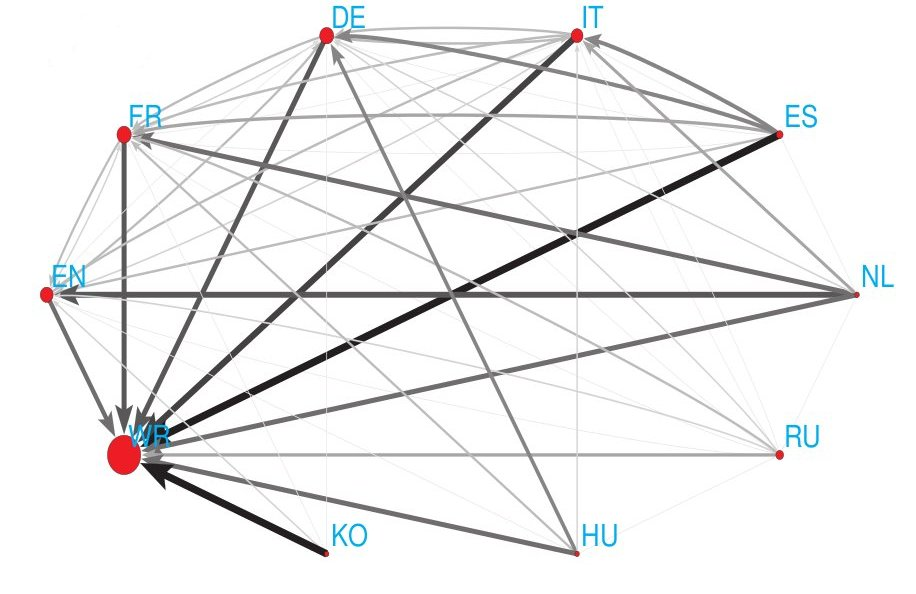Highlighting entanglement of cultures via ranking of multilingual Wikipedia articles
by Y.-H.Eom and D.L.Shepelyansky
arXiv:1306.6259[cs.SI]Left: Network of cultures from PageRank; Right: Google matrix of network of cultures

 ` `Article download: v1 (here); v2 (here); support information (here); published paper + SI (here)Top 30 Wiki personalities in PageRank: EN (here), FR (here), DE (here), IT (here), ES (here), NL (here), RU (here), HU (here), KO (here),Top 30 Wiki personalities in 2DRank: EN (here), FR (here), DE (here), IT (here), ES (here), NL (here), RU (here), HU (here), KO (here),Top 30 Wiki personalities in CheiRank: EN (here), FR (here), DE (here), IT (here), ES (here), NL (here), RU (here), HU (here), KO (here),Top 10000 Wiki articles in PageRank: EN (here), FR (here), DE (here), IT (here), ES (here), NL (here), RU (here), HU (here), KO (here),Top 10000 Wiki articles in 2DRank: EN (here), FR (here), DE (here), IT (here), ES (here), NL (here), RU (here), HU (here), KO (here),Top 10000 Wiki articles in CheiRank: EN (here), FR (here), DE (here), IT (here), ES (here), NL (here), RU (here), HU (here), KO (here),Fig2. gray version (here)Data for fig3 are (a-here), (b-here), (c-here)Data for fig4 are (a-here), (b-here), (c-here)Data for fig6 are (a-here), (b-here)Network data have been generated by Sebastiano Vigna (http://vigna.dsi.unimi.it/) and can be requested from his web page This webpage is created at July 3, 2013 and is maintained by D.L.Shepelyansky